At work I am currently working on estimating/forecasting healthcare spending. Similar to work I have done on forecasting person level crime risks (Wheeler et al., 2019), I build the predictive model dataset like this:

``````CrimeYear2020 PriorCrimeA PriorCrimeB
0              2          3
1              5          0
0              0          0``````

etc. So I flatten people to a single row, and as covariates include prior cumulative crime histories. Most people do this similarly in the healthcare setting, so it looks like:

``````SpendingYear2020 PriorComorbidA PriorComorbidB
3000              1          2
500              3          0
10000              0          0``````

Or sometimes people do a longitudinal dataset, where it is a `spending*year*person` panel (see Lauffenburger et al., 2020 for an example). I find this approach annoying for a few reasons though. One, it requires arbitrary temporal binning, which is somewhat problematic in these transaction level databases. We are talking for chronic offenders a few crimes per year is quite high, and ditto in the healthcare setting a few procedures a year can be very costly. So there is not much data to estimate the underlying function over time.

A second aspect I think is bad is that it doesn’t take into account the recency of the patterns. So the variables on the right hand side can be very old or very new. And with transaction level databases it is somewhat difficult to define how to estimate the lookback – do you consider it normalized by time? The VOID paper I mentioned we evaluated the long term scores, but the PD that does that chronic offender system has two scores – one a cumulative history and another a 90 day history to attempt to deal with that issue (again ad-hoc).

One approach to this issue from marketing research I have seen from very similar types of transactions databases are models to estimate Customer Lifetime Value (Fader et al. 2005). These models in the end generate a dataset that looks like this:

``````Person    RecentMonths  TotalEvents AveragePurchase
A            3             5            \$50
B            1             2           \$100
C            9             8            \$25``````

TotalEvents should be straightforward, RecentMonths just is a measure of the time since the last purchase, and then you have the average value of the purchases. And using just this data, estimates the probability of any future purchases, as well as projects the total value of the future average purchases. So here I use an example of this approach, using the Wolfgang Philly cohort public data. I am not going into the model more specifically (read some of the Bruce Hardie notes to get a flavor).

I have created some python code to follow along and apply these same customer lifetime value estimates to chronic offender data. Most examples of weighting crime harm apply it to spatial areas (Mitchell, 2019; Wheeler & Reuter, 2021), but you can apply it the same to chronic offender lists (Liggins et al., 2019).

# Example Criminal Lifetime Value in Python

First, install the lifetimes python library – Cam’s documentation is excellent and makes the data manipulation/modelling quite simple.

Here I load in the transaction level crime data, e.g. it just have `person A, 1/5/1960, 1000`, where the 1000 is a crime seriousness index created by Wolfgang. Then the lifetimes package has some simple functions to turn our data into the frequency/recency format.

Note that for these models, you drop the first event in the series. To build a model to do train/test, I also split the data into evens before 1962, and use 1962 as the holdout test period.

``````import lifetimes as lt
import pandas as pd

# Just the columns from dataset II
# ID, SeriousScore, Date
df['Date'] = pd.to_datetime(df['Date'])

# Creating the cumulative data
# Having holdout for one year in future
sd = lt.utils.calibration_and_holdout_data(df,'ID','Date',
calibration_period_end='12-31-1961',
observation_period_end='12-31-1962',
freq='M',
monetary_value_col='SeriousScore')

# Only keeping people with 2+ events in prior period
sd = sd[sd['frequency_cal'] > 0].copy()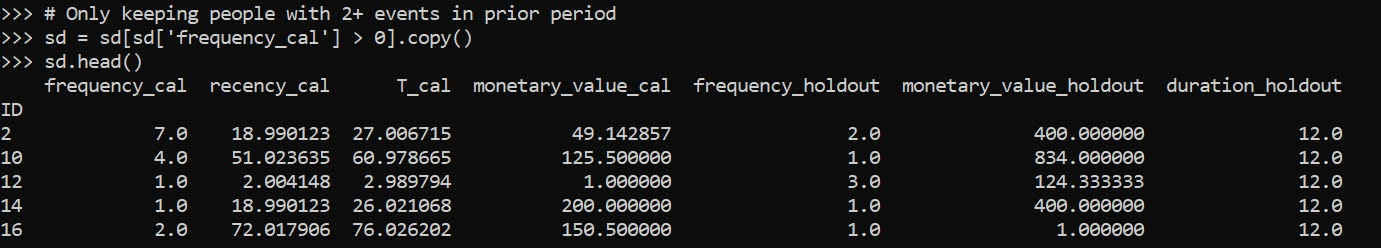Recency_cal is how many months since a prior crime (starting in 1/1/1962), frequency is the total number of events (minus 1, so number of repeat events technically), and the monetary_value_cal here is the average of the crime seriousness across all the events. The way this function works, the variables with the subscript `_cal` are in the training period, and `_holdout` are events in the 1962 period. For subsequent models I subset out people with at least 2 events total in the modeling.

Now we can fit a model to estimate the predicted number of future crimes a person will commit – so this does not take into account the seriousness of those crimes. The final groupby statement shows the predicted number of crimes vs those actually committed, broken down by number of crimes in the training time period. You can see the model is quite well calibrated over the entire sample.

``````# fit BG model
bgf = lt.BetaGeoFitter(penalizer_coef=0)
bgf.fit(sd['frequency_cal'],sd['recency_cal'],sd['T_cal'])

# look at fit of BG model
t = 12
sd['pred_events'] = bgf.conditional_expected_number_of_purchases_up_to_time(t, sd['frequency_cal'], sd['recency_cal'],sd['T_cal'])
sd.groupby('frequency_cal',as_index=False)[['frequency_holdout','pred_events']].sum() # reasonable``````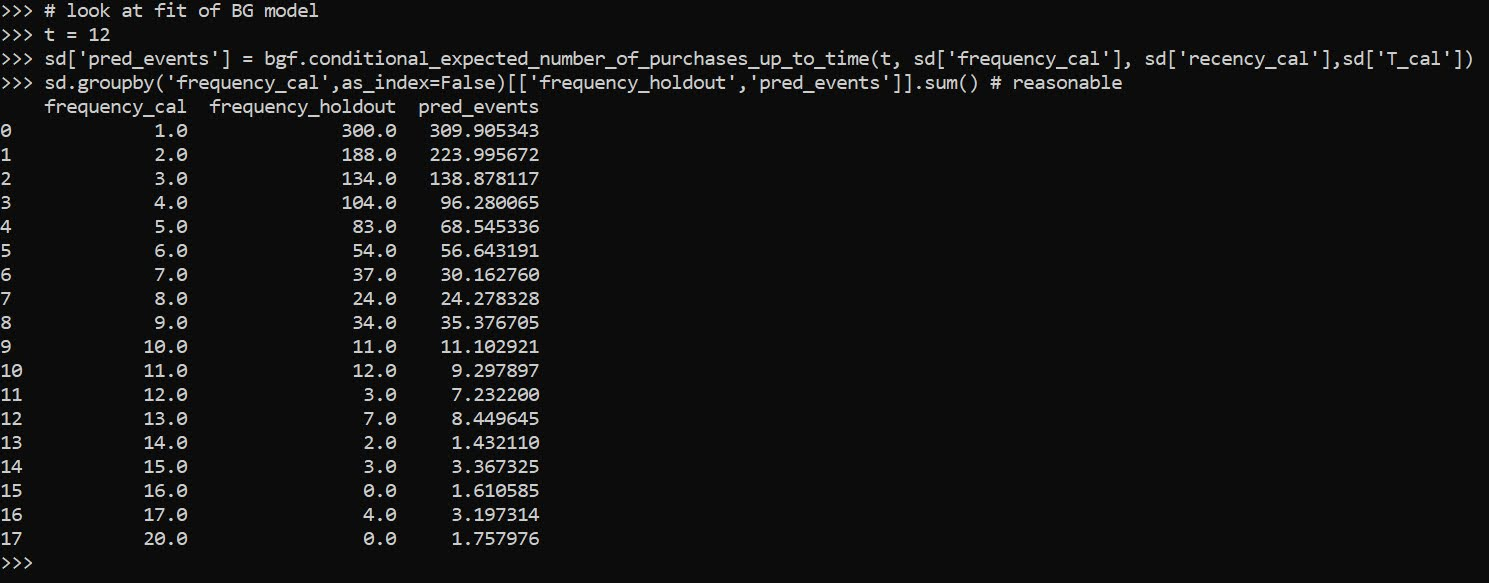Now we can fit a model to estimate the average crime severity score for an individual as well. Then you can project a future cumulative score for an offender (here over a horizon of 1 year), by multiple the predicted number of events times the estimate of the average severity of the events, what I label as `pv` here:

``````# See conditional seriousness
sd['pred_ser'] = ggf.conditional_expected_average_profit(
sd['frequency_cal'],
sd['monetary_value_cal'])

sd['pv'] = sd['pred_ser']*sd['pred_events']
sd['cal_tot_val'] = sd['monetary_value_holdout']*sd['frequency_holdout']
# Not great correlation, around 0.2
vc = ['frequency_holdout','monetary_value_holdout','cal_tot_val','pred_events','pv']
sd[vc].corr()``````

The correlation between `pv` and the holdout cumulative crime severity `cal_tot_val`, is not great at 0.26. But lets look at this relative to the more typical approach analysts will do, simply rank prior offenders based on either total number of events or the crime seriousness:

``````# Lets look at this method via just ranking prior
# seriousness or frequency
sd['rank_freq'] = sd['frequency_cal'].rank(method='first',ascending=True)
sd['rank_seri'] = (sd['monetary_value_cal']*sd['frequency_cal']).rank(method='first',ascending=True)
vc += ['rank_freq','rank_seri']
sd[vc].corr()[vc[-3:]]``````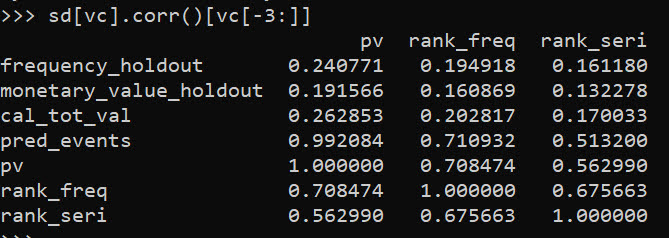So we can see that `pv` outperforms ranking based on total crimes (`rank_freq`), or ranking based on the cumulative serious score for offenders (`rank_seri`) in terms of the correlation for either the total number of future events or the cumulative crime harm.

If we look at capture rates, e.g. pretend we highlight the top 50 chronic offenders for intervention, we can see the criminal lifetime value `pv` estimate outperforms either simple ranking scheme by quite a bit:

``````# Look at capture rates by ranking
topn = 50
res_summ = []
for v in vc[-3:]:
rank = sd[v].rank(method='first',ascending=False)
locv = sd[rank <= topn].copy()
tot_crimes = locv['frequency_holdout'].sum()
tot_ser = locv['cal_tot_val'].sum()
res_summ.append( [v,tot_crimes,tot_ser,topn] )

res_df = pd.DataFrame(res_summ,columns=['Var','TotCrimes','TotSer','TotN'])
res_df``````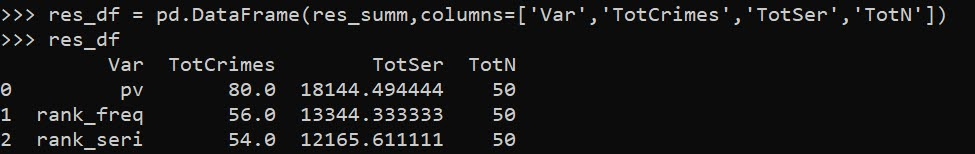In terms of the seriousness projection, it is reasonably well calibrated over the entire sample, but has a very tiny variance – it basically just predicts the average crime serious score over the sample and assigns that as the prediction going forward:

``````# Cumulative stats over sample reasonable
# variance much too small
sd[['cal_tot_val','pv']].describe()``````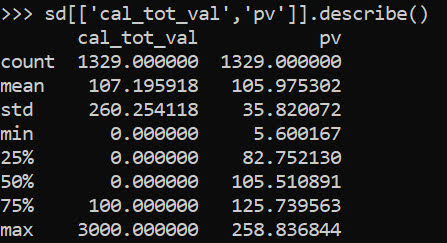So what this means is that if say Chicago READI wanted to do estimates to reasonably justify the max dollar cost for their program (over a large number of individuals) that would be reasonable. And this is how most marketing people use this info, average benefits of retaining a customer.

For individual projections though, e.g. I think OffenderB will generate between `[low,high]` crime harm in the next year, this is not quite up to par. I am hoping though to pursue these models further, maybe either in a machine learning/regression framework to estimate the parameters directly, or to use mixture models in an equivalent way that marketers use “segmentation” to identify different types of customers. Knowing the different way people have formulated models though is very helpful to be able to build a machine learning framework, which you can incorporate covariates.

# A linear programming example for TURF analysis in python

Recently on LinkedIn I saw a very nice example of TURF (Total Unduplicated Reach & Frequency) analysis via Jarlath Quinn. I suggest folks go and watch the video, but for a simple example imagine you are an ice cream food truck, and you only have room in your truck to sell 5 different ice-creams at a time. Some people only like chocolate, others like vanilla and neapolitan, and then others like me like everything. So what are the 5 best flavors to choose to maximize the number of people that like at least one flavor?

You may think this is a bit far-fetched to my usual posts related to criminal justice, but it is very much related to the work I did on identifying optimal gang members to deliver the message in a Focused Deterrence initiative. (I’m wondering if also there is an application in Association Rules/Conjunctive analysis.) But most examples I see of this are in the marketing space, e.g. whether to open a new store, or to carry a new product in a store, or to spend money on ads to reach an audience, etc.

Here I have posted the python code and data used in the analysis, below I go through the steps in formulating different linear programs to tackle this problem. I ended up taking some example simulated data from the XLStat website. (If you have a real data example feel free to share!)

A bit of a side story – growing up in rural Pennsylvania, going out to restaurants was sort of a big event. I specifically remember when we would travel to Williamsport, we would often go to eat at a restaurant called Hoss’s and we would all just order the salad bar buffet. So I am going to pretend this restaurant survey is maximizing the reach for Hoss’s buffet options.

# Frontmatter

Here I am using pulp to fit the linear programming, reading in the data, and I am making up names for the columns for different food items. I have a set of main course meals, sides, and desserts. You will see in a bit how I incorporate this info into the buffet plans.

``````############################################################
#FRONT MATTER

import pandas as pd
import pulp
import os

os.chdir('D:\Dropbox\Dropbox\Documents\BLOG\TURF_Analysis\Analysis')

#This is simulated data from XLStat

#Need 27 total of match up simulated data
main = ['Steak',
'Pizza',
'FriedChicken',
'BBQChicken',
'GrilledSalmon',
'Roast',
'Burger',
'Wings']

'TomatoSoup',
'OnionSoup',
'Peas',
'BrusselSprouts',
'GreenBeans',
'Corn',
'DeviledEggs',
'Pickles']

desserts = ['ChoclateIceCream',
'VanillaIceCream',
'Brownie',
'Blondie',
'CherryPie']

#Renaming columns
surv_data.columns = main + sides + desserts

#Replacing the likert scale data with 0/1
surv_data.replace([1,2,3],0,inplace=True)
surv_data.replace([4,5],1,inplace=True)

#A customer weight example, here setting to 1 for all
surv_data['CustWeight'] = 1
cust_weight = surv_data['CustWeight']
############################################################``````

# Maximizing Customers Reached

And now onto the good stuff, here is an example TURF model linear program. I end up picking the same 5 items that the XLStat program picked in their spreadsheet as well.

``````############################################################

k = 5 #pick 5 items
Cust_Index = surv_data.index
Prod_Index = main + sides + desserts

#Problem and Decision variables
P = pulp.LpProblem("TURF", pulp.LpMaximize)
Cust_Dec = pulp.LpVariable.dicts("Customers Reached", [i for i in Cust_Index], lowBound=0, upBound=1, cat=pulp.LpInteger)
Prod_Dec = pulp.LpVariable.dicts("Products Selected", [j for j in Prod_Index], lowBound=0, upBound=1, cat=pulp.LpInteger)

#Objective Function
P += pulp.lpSum( Cust_Dec[i] * cust_weight[i] for i in Cust_Index )

surv_items = surv_data[Prod_Index] #Dont want the weight variable
#Reached Constraint
for i in Cust_Index:
#Get the products selected
p_sel = surv_items.loc[i] == 1
sel_prod = list(p_sel.index[p_sel])
#Set the constraint
P += Cust_Dec[i] <= pulp.lpSum( Prod_Dec[j] for j in sel_prod )

#Total number of products selected constraint
P += pulp.lpSum( Prod_Dec[j] for j in Prod_Index) == k

#Now solve the model
P.solve()

#Figure out the total reached people
print( pulp.value(P.objective) ) #129

#Print out the products you picked
picked = []
for n,j in enumerate(Prod_Index):
if Prod_Dec[j].varValue == 1:
picked.append( (n+1,j) )

print(picked)

#Same as XLStat
#[(14, 'OnionSoup'), (15, 'Peas'), (16, 'BrusselSprouts'),

#For 5 items, XLStat selected items
# 14 15 16 23 26 that reached 129 people
############################################################``````

One of the things I have done here is to create a ‘weight’ variable associated with each customer. So here I say all of the customers weights are all equal to 1, but you could swap out whatever you wanted. Say you had estimates on how much different individuals spend, so you could give big spenders more weight. (In a criminal justice example, for the Focused Deterrence initiative, folks typically want to target ‘leaders’ more frequently, so you may give them more weight in this example.) Since these examples are based on surveys, you may also want the weight to correspond to the proportion that survey respondent represents in the population, aka raking weights. Or if you have a crazy large survey population, you could use frequency weights for responses that give the exact same picks.

One thing to note as well in this formula is that I recoded the data earlier to be 0/1. You might however consider the likert scale rating 1 to 5 directly, subtract 1 and divide by 4. Then take that weight, and instead of the line:

``Cust_Dec[i] <= pulp.lpSum( Prod_Dec[j] for j in sel_prod )``

You may want something like:

``Cust_Dec[i] <= pulp.lpSum( Prod_Dec[j]*likert_weight[i,j] for j in sel_prod )``

In that case you would want to set the `Cust_i` decision variable to a continuous value, and then maybe cap it at 1 (so you can partially reach customers).

The total number of decision variables will be the number of customers plus the number of potential products, so here only `185 + 27 = 212`. And the number of constraints will be the number of customers plus an additional small number. I’d note you can easily solve systems with 100,000’s of decision variables and constraints on your laptop, so at least for the example TURF analyses I have seen they are definitely within the ‘can solve this in a second on a laptop’ territory.

You can add in additional constraints into this problem. So imagine we always wanted to select one main course, at least two side dishes, and no more than three desserts. Also say you never wanted to pair two items together, say you had two chicken dishes and never wanted both at the same time. Here is how you could do each of those different constraints in the problem.

``````############################################################
#CONSTRAINTS ON ITEMS SELECTED

#Redoing the initial problem, but select 7 items
k = 7
P2 = pulp.LpProblem("TURF", pulp.LpMaximize)
Cust_Dec2 = pulp.LpVariable.dicts("Customers Reached", [i for i in Cust_Index], lowBound=0, upBound=1, cat=pulp.LpInteger)
Prod_Dec2 = pulp.LpVariable.dicts("Products Selected", [j for j in Prod_Index], lowBound=0, upBound=1, cat=pulp.LpInteger)
P2 += pulp.lpSum( Cust_Dec2[i] * cust_weight[i] for i in Cust_Index )
for i in Cust_Index:
p_sel = surv_items.loc[i] == 1
sel_prod = list(p_sel.index[p_sel])
P2 += Cust_Dec2[i] <= pulp.lpSum( Prod_Dec2[j] for j in sel_prod )
P2 += pulp.lpSum( Prod_Dec2[j] for j in Prod_Index) == k

#No Fried and BBQ Chicken at the same time
P2 += pulp.lpSum( Prod_Dec2['FriedChicken'] + Prod_Dec2['BBQChicken']) <= 1
#Exactly one main course
P2 += pulp.lpSum( Prod_Dec2[m] for m in main) == 1
#At least two sides (but could have 0)
P2 += pulp.lpSum( Prod_Dec2[s] for s in sides) >= 2
#No more than 3 desserts
P2 += pulp.lpSum( Prod_Dec2[d] for d in desserts) <= 3

#Now solve the model and print results
P2.solve()
print( pulp.value(P2.objective) ) #137
picked2 = []
for n,j in enumerate(Prod_Index):
if Prod_Dec2[j].varValue == 1:
picked2.append( (n+1,j) )
print(picked2)
#[(10, 'Wings'), (12, 'IcebergSalad'), (14, 'OnionSoup'), (15, 'Peas'),
# (16, 'BrusselSprouts'), (23, 'ChocChipCookie'), (27, 'CherryPie')]
############################################################``````

You could also draw a trade-off curve for how many more people you will reach if you can up the total number of items you can place on the menu, so estimate the model with 4, 5, 6, etc items and see how many more people you can reach if you extend the menu.

One of the other constraints you may consider in this formula is a budget constraint. So imagine instead of the food example, you are working for a marketing company, and you have an advertisement budget. You want to maximize the customer reach given the budget, so here a “product” may be a billboard, radio ad, newspaper ad, etc, but each have different costs. So instead of the constraint `Prod_j == k` where you select so many products, you have the constraint `Prod_j*Cost_j <= Budget`, where each product is associated with a particular cost.

# Alt Formula, Minimizing Cost while Reaching a Set Amount

So in that last bit I mentioned costs for selecting a particular portfolio of products. Another way you may think about the problem is minimizing cost while meeting constraints on the reach (instead of maximizing reach while minimizing cost). So say you were a marketer, and wanted an estimate of how much budget you would need to reach a million people. Or going with our buffet example, imagine we wanted to appeal to at least 50% of our sample (so at least 93 people). Our formula would then be below (where I make up slightly different costs for buffet each of the buffet options).

``````############################################################
#MINIMIZE COST

cost_prod = {'Steak' : 5.0,
'Pizza' : 2.0,
'FriedChicken' : 4.0,
'BBQChicken' : 3.5,
'GrilledSalmon' : 4.5,
'Roast' : 3.9,
'Burger' : 1.5,
'Wings' : 2.4,
'TomatoSoup' : 0.4,
'OnionSoup' : 0.9,
'Peas' : 0.6,
'BrusselSprouts' : 0.5,
'GreenBeans' : 0.4,
'Corn' : 0.3,
'DeviledEggs' : 0.7,
'Pickles' : 0.73,
'ChoclateIceCream' : 1.3,
'VanillaIceCream' : 1.2,
'Brownie' : 1.2,
'Blondie' : 1.3,
'CherryPie' : 1.9}

#Setting up the model with the same selection constraints
n = 100
Pmin = pulp.LpProblem("TURF", pulp.LpMinimize)
Cust_Dec3 = pulp.LpVariable.dicts("Customers Reached", [i for i in Cust_Index], lowBound=0, upBound=1, cat=pulp.LpInteger)
Prod_Dec3 = pulp.LpVariable.dicts("Products Selected", [j for j in Prod_Index], lowBound=0, upBound=1, cat=pulp.LpInteger)
#Minimize this instead of Maximize reach
Pmin += pulp.lpSum( Prod_Dec3[j] * cost_prod[j] for j in Prod_Index )
for i in Cust_Index:
p_sel = surv_items.loc[i] == 1
sel_prod = list(p_sel.index[p_sel])
Pmin += Cust_Dec3[i] <= pulp.lpSum( Prod_Dec3[j] for j in sel_prod )
#Instead of select k items, we want to reach at least n people
Pmin += pulp.lpSum( Cust_Dec3[i]*cust_weight[i] for i in Cust_Index) >= n

#Same constraints on meal choices
Pmin += pulp.lpSum( Prod_Dec3['FriedChicken'] + Prod_Dec3['BBQChicken']) <= 1
Pmin += pulp.lpSum( Prod_Dec3[m] for m in main) == 1
Pmin += pulp.lpSum( Prod_Dec3[s] for s in sides) >= 2
Pmin += pulp.lpSum( Prod_Dec3[d] for d in desserts) <= 3

#Now solve the model and print results
Pmin.solve()

reached = 0
for i in Cust_Index:
reached += Cust_Dec3[i].varValue
print(reached) #100 reached on the nose

picked = []
for n,j in enumerate(Prod_Index):
if Prod_Dec3[j].varValue == 1:
picked.append( (n+1,j,cost_prod[j]) )
cost += cost_prod[j]
print(picked)
#[(9, 'Burger', 1.5), (13, 'TomatoSoup', 0.4), (15, 'Peas', 0.6)]
print(pulp.value(Pmin.objective)) #Total Cost 2.5
############################################################``````

So for this example our minimum budget buffet has some burgers, tomato soup, and peas. (Sounds good to me, I am not a picky eater!)

You can still incorporate all of the same other constraints I discussed before in this formulation. So here we need at a minimum to serve only 3 items to get the (over) 50% reach that we desire. If you wanted fairness type constraints, e.g. you want to reach 60% of females and 40% of males, you could do that as well. In that case you would just have two separate constraints for each group level you wanted to reach (which would also be applicable to the prior maximize reach formula, although you may need to keep upping the number of products selected before you identify a feasible solution).

In the end you could mash up these two formulas into one bi-objective function. You would need to define a term though to balance reach and cost. I’m wondering as well if there is a way to incorporate marginal benefits of sales into this as well, e.g. if you sell a Steak you may make a larger profit than if you sell a Pizza. But I am not 100% sure how to do that in this set up (even though I like all ice-cream, I won’t necessarily buy every flavor if I visit the shop). Similar for marketing adverts some forms may have better reach, but may have worse conversion rates.